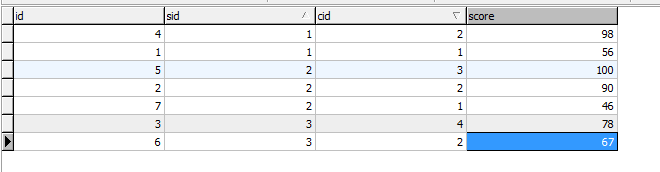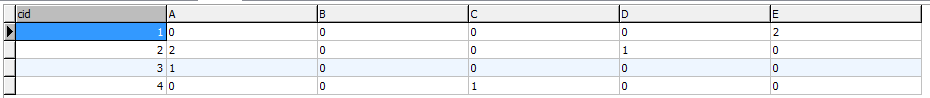# 统计出每门课程各个成绩等级的学生数量

SQL语句如下：

select  cid,
sum(case when score>=90 and score<=100 then 1 else 0 end) A,
sum(case when score>=80  and score<90 then 1 else 0 end) B,
sum(case when score>=70  and score<80 then 1 else 0 end) C,
sum(case when score>=60  and score<70 then 1 else 0 end) D,
sum(case when score<60 then 1 else 0 end) E
from sc
group by cid©️2019 CSDN 皮肤主题: 大白 设计师: CSDN官方博客